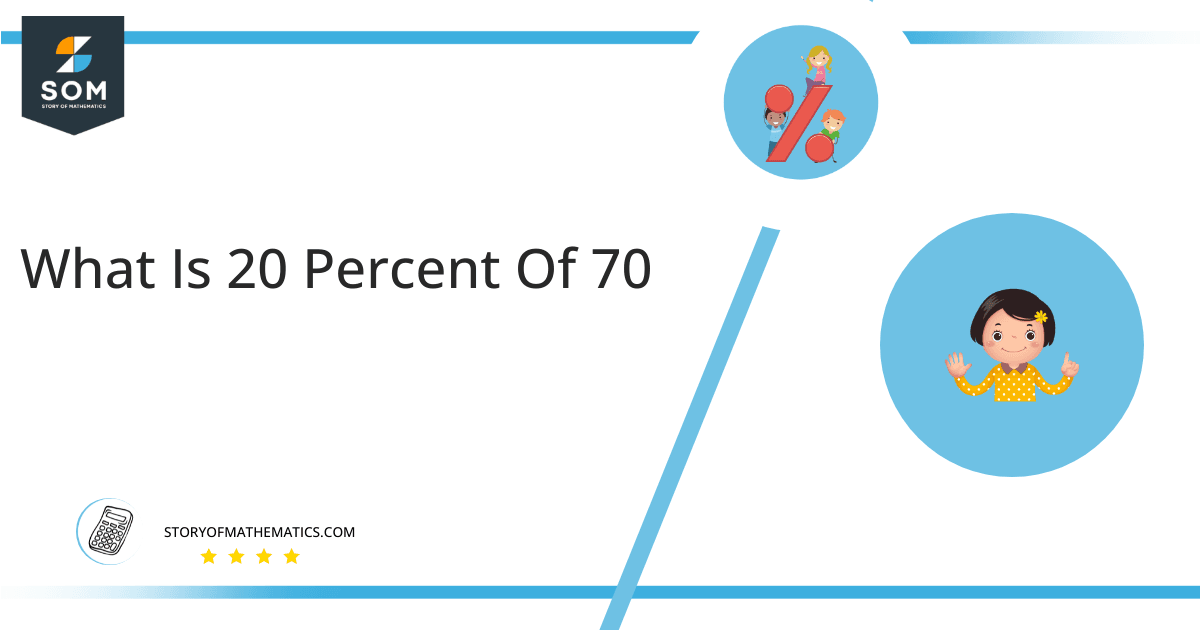# What is 20 percent of 70 + Solution with Free Steps20 percent of 70 is equivalent to 14. The factor 0.20 multiplied by 70 will give this answer.

Sometimes the information given to you might not be as simple as it looks. Picture a situation where you act as a teacher and you wish to know how many girls are there in the class if there are 20% girls and the rest are boys for a total of 70 students. In order to correctly keep track of your students, you must now calculate 20% of 70. Keeping in view our brief assumption, you have 14 girls as of now, and 56 boys making a total of 70 students. Such problems are known to be quite useful in daily routines.

Except for this specific solution, an in-depth illustration is given below which will help you to understand how we calculate 20 percent of 70.

## What is 20 percent of 70?

The 20 percent of 70 is equal to the number 14. To get this answer, just multiply the fraction 0.20 by the number 70.

The answer can be acquired by taking the fraction 20/100 and multiplying it by 70. When you solve the equation the final solution will come out to be 14.

## How To Calculate 20 percent of 70?

The simplest way to estimate 20 percent of 70 is by using basic mathematical computation.  The following steps must be followed to calculate the 20 percent of 70.## Step 1

Firstly, describe 20 percent of 70 in terms of a mathematical phrase:

20 percent of 70 = 20% x 70

## Step 2

We know that percentage sign % is equal to 1/100.

Use the above equation thus,

20 percent of 70 = (20 /100) x 70

## Step 3

Next, simplifying the above equation gives:

20 percent of 70 = (20 x 70) / 100

## Step 4

Cutting the common terms:

20 percent of 70 = (2 x 70) / 10

## Step 5

Upon further solving and simplifying the above equation we get the final answer:

20 percent of 70 = (2 x 7) = 14

Therefore, 20 percent of 70 is equivalent to 14.

We can see that 20 percent of 70 is equal to 14 in the form of a pie chart:In the above Chart, the orange part shows 20% of 70. The circle is of 70 portions out of which 20 percent is highlighted. The remainder of the 80% is equivalent to 56 as 70 – 14 = 56. This is displayed by the green portion.

All the Mathematical drawings/images are created using GeoGebra.